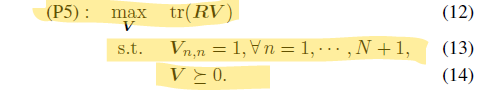# Semidefinite relaxation problem

Hello,

I am attempting to solve the following semidefinite program:The code that I employ reads as follows:

cvx_begin
variables V(N+1,N+1) R(N+1,N+1)
diag(V) == 1
V == semidefinite(N+1)
maximize(trace(R*V))
cvx_end

However, I receive the following error:

Error using * (line 126)
Disciplined convex programming error:
Only scalar quadratic forms can be specified in CVX
.

Error in SDR (line 9)
maximize(trace(R*V))

Any help would be appreciated. Thank you!

1 Like

Apparently `R` is input data, but you have declared it as a variable. Do not declare `R` as a variable. It should be a numerically populated matrix prior to its use in your CVX program.

1 Like